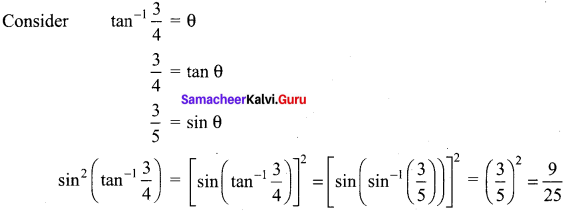# Samacheer Kalvi 12th Maths Solutions Chapter 4 Inverse Trigonometric Functions Ex 4.3

## Tamilnadu Samacheer Kalvi 12th Maths Solutions Chapter 4 Inverse Trigonometric Functions Ex 4.3

Question 1.
Find the domain of the following functions:
(i) $$\tan ^{-1}(\sqrt{9-x^{2}})$$
(ii) $$\frac{1}{2} \tan ^{-1}\left(1-x^{2}\right)-\frac{\pi}{4}$$
Solution:
(i) f(x) = $$\tan ^{-1}(\sqrt{9-x^{2}})$$
We know the domain of tan-1 x is (-∞, ∞) and range is $$\left(-\frac{\pi}{2}, \frac{\pi}{2}\right)$$
So, the domain of f(x) = $$\tan ^{-1}(\sqrt{9-x^{2}})$$ is the set of values of x satisfying the inequality
$$-\infty \leq \sqrt{9-x^{2}} \leq \infty$$
⇒ 9 – x2 ≥ 0
⇒ x2 ≤ 9
⇒ |x| ≤ 3
Since tan x is an odd function and symmetric about the origin, tan-1 x should be an increasing function in its domain.
∴ Domain is (2n + 1)$$\frac{\pi}{2}$$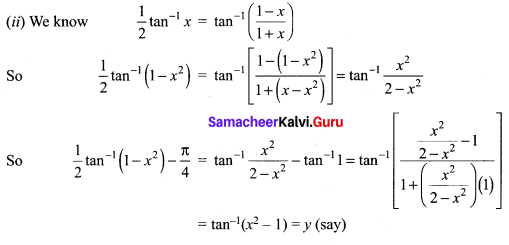The domain of y is (-∞, ∞) {x | x ∈ -1} and range is [-1, ∞) {y | y ≥ -1}
The domain for tan-1(x2 – 1) is (2n + 1)π. Since tan x is an odd function.

Question 2.
Find the value of
(i) $$\tan ^{-1}\left(\tan \frac{5 \pi}{4}\right)$$
(ii) $$\tan ^{-1}\left(\tan \left(-\frac{\pi}{6}\right)\right)$$
Solution: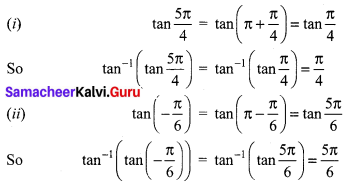Question 3.
Find the value of
(i) $$\tan \left(\tan ^{-1} \frac{7 \pi}{4}\right)$$
(ii) tan(tan-1(1947))
(iii) tan(tan-1(-0.2021))
Solution:
We know that tan(tan-1 x) = x
(i) $$\tan \left(\tan ^{-1} \frac{7 \pi}{4}\right)=\frac{7 \pi}{4}$$
(ii) tan(tan-1(1947))= 1947
(iii) tan(tan-1 (-0.2021)) = -0.2021

Question 4.
Find the value of
(i) $$\tan \left(\cos ^{-1}\left(\frac{1}{2}\right)-\sin ^{-1}\left(-\frac{1}{2}\right)\right)$$
(ii) $$\sin \left(\tan ^{-1}\left(\frac{1}{2}\right)-\cos ^{-1}\left(\frac{4}{5}\right)\right)$$
(iii) $$\cos \left(\sin ^{-1}\left(\frac{4}{5}\right)-\tan ^{-1}\left(\frac{3}{4}\right)\right)$$
Solution: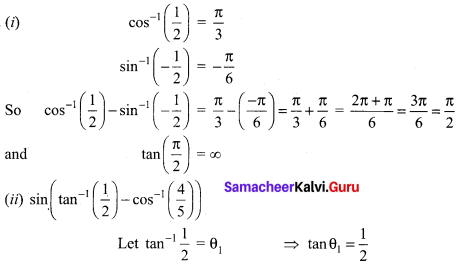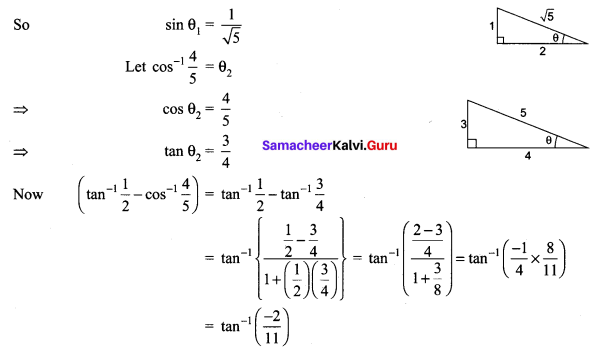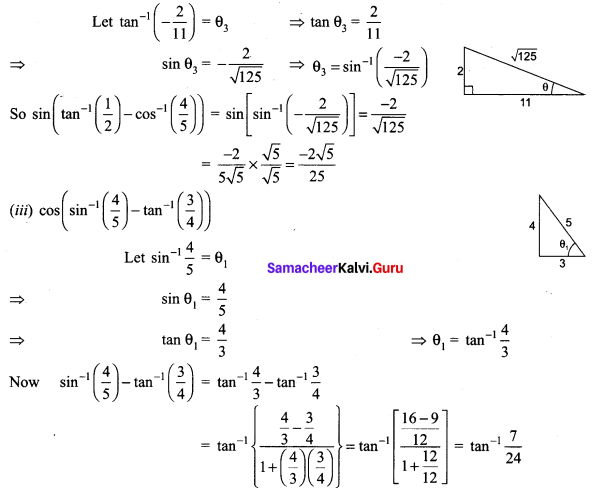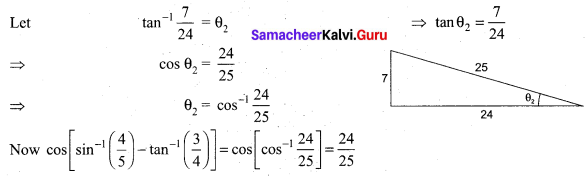### Samacheer Kalvi 12th Maths Solutions Chapter 4 Inverse Trigonometric Functions Ex 4.3 Additional Problems

Question 1.
Find the principle value of: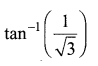Solution: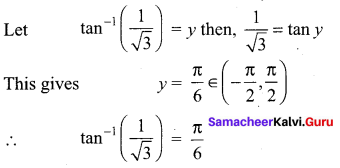Question 2.
Find the value of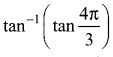Solution: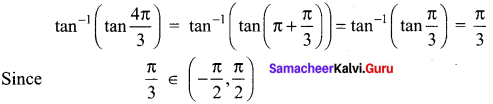Question 3.
Find the value of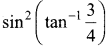Solution: